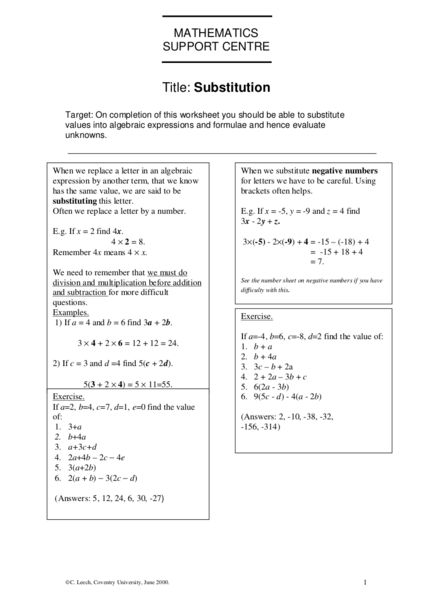# Substitution into Algebraic ExpressionsContributed by:This pdf covers the basics of substitution into algebraic expressions with examples for better understanding.
1. MATHEMATICS
SUPPORT CENTRE
Title: Substitution
Target: On completion of this worksheet you should be able to substitute
values into algebraic expressions and formulae and hence evaluate
unknowns.
When we replace a letter in an algebraic When we substitute negative numbers
expression by another term, that we know for letters we have to be careful. Using
has the same value, we are said to be brackets often helps.
substituting this letter.
Often we replace a letter by a number. E.g. If x = -5, y = -9 and z = 4 find
3x - 2y + z.
E.g. If x = 2 find 4x.
4 × 2 = 8. 3×(-5) - 2×(-9) + 4 = -15 – (-18) + 4
Remember 4x means 4 × x. = -15 + 18 + 4
= 7.
We need to remember that we must do
division and multiplication before addition See the number sheet on negative numbers if you have
and subtraction for more difficult difficulty with this.
Examples. Exercise.
1) If a = 4 and b = 6 find 3a + 2b.
If a=-4, b=6, c=-8, d=2 find the value of:
3 × 4 + 2 × 6 = 12 + 12 = 24. 1. b + a
2. b + 4a
2) If c = 3 and d =4 find 5(c + 2d). 3. 3c – b + 2a
4. 2 + 2a – 3b + c
5(3 + 2 × 4) = 5 × 11=55. 5. 6(2a - 3b)
Exercise. 6. 9(5c - d) - 4(a - 2b)
If a=2, b=4, c=7, d=1, e=0 find the value
of: (Answers: 2, -10, -38, -32,
1. 3+a -156, -314)
2. b+4a
3. a+3c+d
4. 2a+4b – 2c − 4e
5. 3(a+2b)
6. 2(a + b) − 3(2c − d)
(Answers: 5, 12, 24, 6, 30, -27)
C. Leech, Coventry University, June 2000. 1
2. When letters are multiplied together or divided Substitution is frequently used with formulae. A
by each other the same principles apply. To formula expresses one variable in terms of other
avoid mistakes with minus signs we should variables.
again use brackets. E.g. V = IR, is a formula.
E.g. If a = 2, b = -6, and c=5 find the value of
We are often told the values of some of the
4ab + 7c 2 .
variables and asked to find the value of the others.
4 × 2 × (-6) + 7 × 5 2 = -48 + 175 E.g. If V = IR what is the value of V when I = 15
=127. when R = 16.
Remember 4ab means 4×a×b. V = 15×16 = 240.
Exercise.
If a = 5, b = -6, c = 2, d = -10 find the value of
1. If S = UV and U=5 and V=12 find S.
1. ac
b
2. ab - d 2 2. If H = and b=4 and c=8 find H.
3. 3(c - 4a) c
c rs
4. 3. If p= 2 and r = 4, s=12 and q=4 find p.
d q
ab (Answers: 60, 1 , 3)
5. 2
d −a
(Answers: 10, -130, -120, - 1 , 2)
5
C. Leech, Coventry University, June 2000. 2